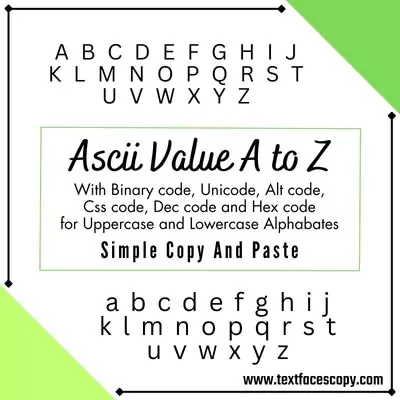# Ascii Value Of Q Copy And Paste

(｀ᴗ´)っ Click on any Text to copy !!!

Copied

Are you searching for ascii value of Q ? then you are right place. Here you get ascii code for Q uppercase and lowercase, binary code for Q, alt code for Q, unicode, css code, dec code and hex code for Q.

## Ascii Value Of Q (Uppercase)

ASCII Code 81 01010001 U+0051 81 \0051 Q Q

## Ascii Value Of q (Lowercase)

ASCII Code 113 01110001 U+0071 113 \0071 q q## What is ascii value ?

ASCII (American Standard Code for Information Interchange) is a character encoding standard that assigns a unique numerical value (between 0 and 127) to each character used in the English language, including letters, digits, punctuation marks, and control characters. The ASCII value of a character is the decimal value that corresponds to that character in the ASCII table. For example, the ASCII value of the letter "A" is 65, the ASCII value of the digit "0" is 48, and the ASCII value of the punctuation mark "?" is 63. The ASCII standard is widely used in computing to represent text data and allows different computers and devices to exchange information in a standardized way.

## How to find ascii value of any symbol and character ?

To find the ASCII value of any symbol, you can use the built-in ord() function in Python. The ord() function returns the integer representing the Unicode character. Since ASCII is a subset of Unicode, this function can be used to find the ASCII value of a symbol as well.

## What is the ascii value of lowercase and uppercase q ?

The ASCII value of lowercase "q" is 113 and the ASCII value of uppercase "Q" is 81.

## How are ASCII values used in programming ?

In programming, ASCII values are often used to represent characters as numerical values. This allows computers to store and manipulate textual data in a standardized way. The ASCII value of lowercase "q" (i.e., 113) can be used in various operations on characters, such as checking if a character is a consonant, converting uppercase to lowercase or vice versa, or performing string operations.

Do you want to see ascii art ? then click here for more than 5000 different ascii art with one click copy and paste.

End of content

ᕙ[◣益◢]ᕗ
Angry
(ᵔᴥᵔ)
Happy
(◞╭╮◟)
(˃̣̣̥⌓˂̣̣̥)
Cry
(♥ﻬ♥)
Love
|◙‿◙|
Cute
(ꈍ .̮ ꈍ)
Shy
(˘ε˘˶ )
Kiss
(〴ᴗ˘)〴
Flirty
(˵´• ε •`˵)
Blushing
(o_O")
Confused